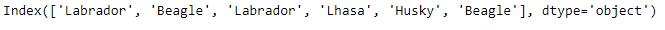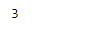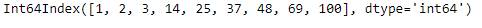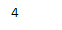Related Articles
Python | Pandas Index.get_loc()
• Last Updated : 17 Dec, 2018

Python is a great language for doing data analysis, primarily because of the fantastic ecosystem of data-centric python packages. Pandas is one of those packages and makes importing and analyzing data much easier.

Pandas` Index.get_loc()` function return integer location, slice or boolean mask for requested label. The function works with both sorted as well as unsorted Indexes. It provides various options if the passed value is not present in the Index. you may choose to return the previous value or the next value to the passed value only if the Index labels are sorted.

Syntax: Index.get_loc(key, method=None, tolerance=None)

Parameters:
key : label
method : {None, ‘pad’/’ffill’, ‘backfill’/’bfill’, ‘nearest’}, optional
-> default: exact matches only.
-> pad / ffill: find the PREVIOUS index value if no exact match.
-> backfill / bfill: use NEXT index value if no exact match
-> nearest: use the NEAREST index value if no exact match. Tied distances are broken by preferring the larger index value.

Returns : loc : int if unique index, slice if monotonic index, else mask

Example #1: Use `Index.get_loc()` function to find the location of the passed value.

 `# importing pandas as pd ` `import` `pandas as pd ` ` `  `# Creating the Index ` `idx ``=` `pd.Index([``'Labrador'``, ``'Beagle'``, ``'Labrador'``, ` `                     ``'Lhasa'``, ``'Husky'``, ``'Beagle'``]) ` ` `  `# Print the Index ` `idx `

Output :let’s find out the location of ‘Lhasa’ in the Index.

 `# Print the location of the passed value.. ` `idx.get_loc('Lhasa) `

Output :As we can see in the output, the `Index.get_loc()` function has returned 3 indicating that the passed value is present at this location in the Index.

Example #2: Use `Index.get_loc()` function to find the location of the passed value. If the passed value is not present in the Index then return the location of previous value that is just smaller than the passed value.

 `# importing pandas as pd ` `import` `pandas as pd ` ` `  `# Creating the Index ` `idx ``=` `pd.Index([``1``, ``2``, ``3``, ``14``, ``25``, ``37``, ``48``, ``69``, ``100``]) ` ` `  `# Print the Index ` `idx `

Output :Let’s find the position of the value 33 in the Index.

 `# Find the position of 33 in the index. ` `# If it is not present then we forward  ` `# fill and return the position of previous value. ` `idx.get_loc(``33``, method ``=``'ffill'``) `

Output :As we can see the function has returned the output 3. As 33 is not present in the Index but in the sorted order the value that is just smaller than 33 is 25 and its location is 4. So, the output is 4. we do not set method parameter then it will result into error if the value is not present.

Attention geek! Strengthen your foundations with the Python Programming Foundation Course and learn the basics.

To begin with, your interview preparations Enhance your Data Structures concepts with the Python DS Course.

My Personal Notes arrow_drop_up
Recommended Articles
Page :